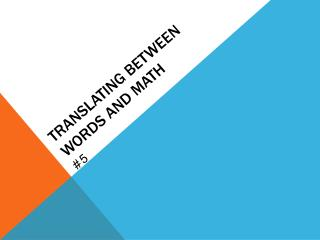Download PresentationTranslating between words and mathTranslating between words and math - PowerPoint PPT Presentation

Download PresentationTranslating between words and math
An Image/Link below is provided (as is) to download presentation

Download Policy: Content on the Website is provided to you AS IS for your information and personal use and may not be sold / licensed / shared on other websites without getting consent from its author. While downloading, if for some reason you are not able to download a presentation, the publisher may have deleted the file from their server.

- - - - - - - - - - - - - - - - - - - - - - - - - - - E N D - - - - - - - - - - - - - - - - - - - - - - - - - - -
Presentation Transcript

1. In word problems, you may need to identify the action to translate words into math. Put together or combine Add Find how much more or less Subtract Put together groups of equal parts Multiply Separate into equal groups Divide

2. Real-World Application: Example 1 Lake Superior is the largest lake in North America. Let a stand for the area in square miles of Lake Superior. Lake Erie has an area of 9,910 square miles. Write an expression to show how much larger Lake Superior is than Lake Erie.

3. Example 2 Let p represent the number of colored pencils in a box. If there are 26 boxes on the shelf, write an algebraic expression to represent the total number of pencils on the shelf.

4. Example 3 The salary of the governor of Florida for the 2008–2009 fiscal year is \$132,932. This is d dollars more than Leones makes as a landscaper. Write an expression for Leones’ salary.

5. Example 4 A group of n friends go to the movies. They purchase adult tickets for \$38. Write an expression for the price of one adult ticket.

6. Example 5 Beth has a pet cat named Buddy. In 3 years, Buddy will be b years old. Beth got Buddy when he was 2 years old. Write an expression that represents how many years Beth has had Buddy. b - 5

7. + Operation Numerical Expression Words Algebraic Expression Words 37 + 28 • 28 added to 37 • 37 plus 28 • the sum of 37 and 28 • 28 more than 37 There are several different ways to write math expressions with words. x+ 28 • 28 added to x • x plus 28 • the sum of x and 28 • 28 more thanx

8. Operation Numerical Expression 90 — 12 • 12 subtracted from 90 • 90 minus 12 • the difference of 90 and 12 • 12 less than 90 • take away 12 from 90 Words Algebraic Expression k— 12 • 12 subtracted from k • k minus 12 • the difference of k and 12 • take away 12 from k Words

9. Operation Numerical Expression 8  48 or 8  48 or (8)(48) or 8(48) or (8)48 • 8 times 48 • 48 multiplied by 8 • the product of 8 and 48 Words Algebraic Expression 8 wor(8)(w)or8w • 8 timesw • w multiplied by 8 • the product of 8 and w • 8 groups ofw Words

10. 327 3 n 3  Operation 327  3 or Numerical Expression • 327 divided by 3 • the quotientof 327 and 3 Words n  3 or Algebraic Expression Words • ndivided by 3 • thequotient of n and 3

11. Example 6: Translating Words into Math Write each phrase as a numerical or algebraic expression. A. 987 minus 12 B. x times 45

12. Example 7 Write each phrase as a numerical or algebraic expression. A. 312 plus 58 B. the quotient of 21 and 10

13. Example 8: Translating Math into Words Write two phrases for each expression. A. 16 __ b B. (75)(32)